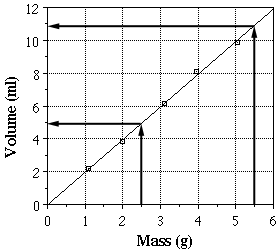# INTERPOLATION AND EXTRAPOLATION

8. INTERPOLATION AND EXTRAPOLATION OF STATIONARY
RANDOM SEQUENCES *
Spectral conditions are established for the possibility of extrapolating and interpolating stationary random sequences by a sufficiently large number of terms
with any prescribed accuracy.
Introduction
For each integer t (-00 < t < +00) let x(t) be areal random variable whose
square has finite expectation. The sequence of random variables x(t) will be
called stationary if the expectations 1
m = Ex(t)
and
B(k) = E[(x(t + k) – m)(x(t) – m)]
do not depend on t. Without loss of generality we may set
m = Ex(t) = o. (1)
Then
B(k) = E[x(t + k)x(t)]. (2)

Since
B(-k) = B(k), (3)
it suffices to consider the second moments B(k) only for k ~ o.
The problem of linear extrapolation of a stationary sequence satisfying (1)
is to select for given n > 0 and m ~ 0 real coefficients a, for which the linear
combination
of random variables
x(t -1), x(t – 2), … ,x(t – n)
gives the closest possible approximation to the random variable z( t + m). It is
natural to take the expectation
n n n
(T2 = E(z(t + m) – L)2 = B(O) – 2 I:B(m + s)a. + I: I: B(p – q)apaq
p=lq=l
as the measure of accuracy of such an approximation.
If the second moments B(k) are known, then it is easy to find the coeffidents a. for which (T2 takes the smallest value. This smallest value of (T2 will
be denoted by (T~ (n, m).
Clearly, (T~ (n, m) cannot increase with n. Therefore the limit
lim (T~(n, m) = (T~(m) n-oo (4)
exists. To find this limit is the first problem we solve in this paper.
As for the interpolation problem, we will consider only the case of estimating z(t) from
z(t + 1), z(t + 2), … , z(t + n),
z(t – 1), z(t – 2), … , z(t – n).
For this case, let (TJ(n) be the minimal expectation

For this case, let (TJ(n) be the minimal expectation
where Q is a linear form
Q = alz(t + 1) + a2z(t + 2) + … + anz(t + n)+
+ a_lz(t – 1) + a_2z(t – 2) + … + a_nz(t – n)
with constant real coeffidents a •.
Since (TJ(n) does not increase with n, the limit
exists.
(5)
Our second goal is to determine (TJ. The solution to both these problems
was announced in my paper .2 It uses notions relating to the spectral theory
of stationary random processes.
2 There is amisprint in formula (1) of . Th, correct form of (1) is:
lim tTJ(n) = r : 1′” d(~).

Besides being able to show trends between variables, plotting data on a graph allows us to predict values for which we have taken no data. When we predict values that fall within the range of data points taken it is called interpolation. When we predict values for points outside the range of data taken it is called extrapolation. Extrapolation over too far a range can be dangerous unless it is certain that the relationship between the variables continues over the entire range.

Consider these examples based on the volume/mass data from the previous page. We could use our graph to interpolate the volume for a sample with a mass of 2.5 g. This is done by drawing a vertical line from the x-axis at a value of 2.5 g until it crosses our best fit line, and then drawing a horizontal line to the y-axis. The y-value at this point, 4.9 ml, is equal to the volume of 2.5 g sample. The same process is used for extrapolation. A sample with a mass of 5.5 g, will have a volume of 10.8 ml.These values could also be determined using the equation for the best fit line determined previously.

volume = 1.982 (mass) + 0.002

If 2.5 g is substituted for the mass, the calculated volume will be 4.96 ml. If a mass of 5.5 g is used, the volume will be 10.90 ml. Both of these values are close to those read off the graph.

Note that while in both of the above examples we determined the volume from a given mass, the opposite could also be performed. If a sample has a volume of 7.0 ml, we could determine that its mass should be 3.53 g.

## STEM Elearning

We at FAWE have built this platform to aid learners, trainers and mentors get practical help with content, an interactive platform and tools to power their teaching and learning of STEM subjects, more

#### How to find your voice as a woman in Africa

© FAWE, Powered by: Yaaka DN.